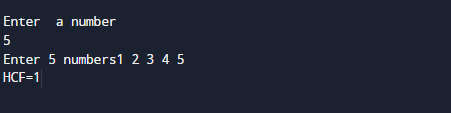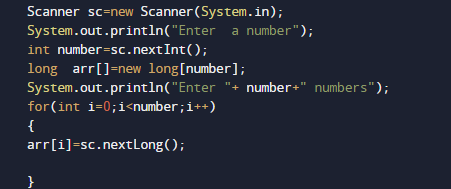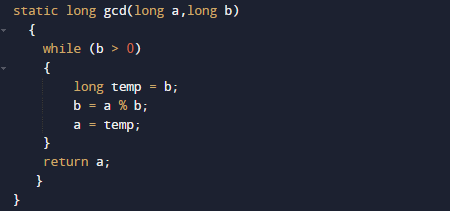# Java Program to Find GCD of N Numbers

In this tutorial you will learn about the Java Program to Find GCD of N Numbers and its application with practical example.

In this tutorial, we will learn to create a Java Program to Find GCD of  N number using Java programming.

## Prerequisites

Before starting with this tutorial we assume that you are best aware of the following Java programming topics:

• Java Operators.
• Basic Input and Output function in Java.
• Class and Object in Java.
• Basic Java programming. Basic Java programming.
• If-else statements in Java.
• For loop in Java.

What Is GCD of HCF?

HCF stands for Highest Common Factor or (GCD) Greatest common divisor .When  we finding the factor of two numbers, Highest Common Factor of  numbers is the Largest factor that divides these two numbers. The greatest factor found from the common factors is called the GCD=> “is the largest positive integer that divides each of the integers“.

## Java Program to Find GCD of N Numbers

In this program we will find GCD of N given number using For loop. We would first declared and initialized the required variables. Next, we would prompt user to input size of an array Later we will find the GCD or  HCF of given numbers.

## OutputIn the above program, we have first declared and initialized a set variables required in the program.

• number =It will hold size of an array elements
• arr[] =It will hold N number given by user.
• i = For iteration.## Let’s see the steps of finding gcd.

* Read the data from user using scanner class method and store those entered values in to the variables arr[].

• Now pass the arr[] and result variable to the static method gcd(result,arr[i]) to find GCD.
• Where within the function gcd() we will find the greatest common divisor of given values and return the GCD.In this tutorial we have learn about the Java Program to Find GCD of N Numbers and its application with practical example. I hope you will like this tutorial.